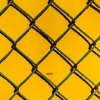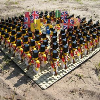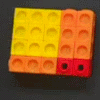# Creating and Manipulating Linear and Quadratic Expressions: Age 14-16

This is part of our Secondary Curriculum collection of favourite rich tasks arranged by topic.### Finding Factors

##### Age 14 to 16Challenge Level

Can you find the hidden factors which multiply together to produce each quadratic expression?### Hollow Squares

##### Age 14 to 16Challenge Level

Which armies can be arranged in hollow square fighting formations?##### Age 14 to 16Challenge Level

Can you find out what is special about the dimensions of rectangles you can make with squares, sticks and units?### Pair Products

##### Age 14 to 16Challenge Level

Choose four consecutive whole numbers. Multiply the first and last numbers together. Multiply the middle pair together. What do you notice?### What's Possible?

##### Age 14 to 16Challenge Level

Many numbers can be expressed as the difference of two perfect squares. What do you notice about the numbers you CANNOT make?### Puzzling Place Value

##### Age 14 to 16Challenge Level

Can you explain what is going on in these puzzling number tricks?### Why 24?

##### Age 14 to 16Challenge Level

Take any prime number greater than 3 , square it and subtract one. Working on the building blocks will help you to explain what is special about your results.### Always Perfect

##### Age 14 to 18Challenge Level

Show that if you add 1 to the product of four consecutive numbers the answer is ALWAYS a perfect square.### Pythagoras Perimeters

##### Age 14 to 16Challenge Level

If you know the perimeter of a right angled triangle, what can you say about the area?### Perfectly Square

##### Age 14 to 16Challenge Level

The sums of the squares of three related numbers is also a perfect square - can you explain why?### Difference of Two Squares

##### Age 14 to 16Challenge Level

What is special about the difference between squares of numbers adjacent to multiples of three?### Multiplication Square

##### Age 14 to 16Challenge Level

Pick a square within a multiplication square and add the numbers on each diagonal. What do you notice?### Plus Minus

##### Age 14 to 16Challenge Level

Can you explain the surprising results Jo found when she calculated the difference between square numbers?### Square Number Surprises

##### Age 14 to 16Challenge Level### Harmonic Triangle

##### Age 14 to 16Challenge Level

Can you see how to build a harmonic triangle? Can you work out the next two rows?### 2-digit Square

##### Age 14 to 16Challenge Level

A 2-Digit number is squared. When this 2-digit number is reversed and squared, the difference between the squares is also a square. What is the 2-digit number?### Creating and Manipulating Linear and Quadratic Expressions - Short Problems

##### Age 11 to 16

A collection of short problems on creating algebraic expressions.You may also be interested in this collection of activities from the STEM Learning website, that complement the NRICH activities above.